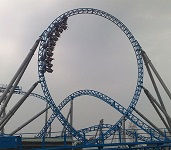# 03: Two-Dimensional Kinematics

$$\newcommand{\vecs}{\overset { \rightharpoonup} {\mathbf{#1}} }$$ $$\newcommand{\vecd}{\overset{-\!-\!\rightharpoonup}{\vphantom{a}\smash {#1}}}$$$$\newcommand{\id}{\mathrm{id}}$$ $$\newcommand{\Span}{\mathrm{span}}$$ $$\newcommand{\kernel}{\mathrm{null}\,}$$ $$\newcommand{\range}{\mathrm{range}\,}$$ $$\newcommand{\RealPart}{\mathrm{Re}}$$ $$\newcommand{\ImaginaryPart}{\mathrm{Im}}$$ $$\newcommand{\Argument}{\mathrm{Arg}}$$ $$\newcommand{\norm}{\| #1 \|}$$ $$\newcommand{\inner}{\langle #1, #2 \rangle}$$ $$\newcommand{\Span}{\mathrm{span}}$$ $$\newcommand{\id}{\mathrm{id}}$$ $$\newcommand{\Span}{\mathrm{span}}$$ $$\newcommand{\kernel}{\mathrm{null}\,}$$ $$\newcommand{\range}{\mathrm{range}\,}$$ $$\newcommand{\RealPart}{\mathrm{Re}}$$ $$\newcommand{\ImaginaryPart}{\mathrm{Im}}$$ $$\newcommand{\Argument}{\mathrm{Arg}}$$ $$\newcommand{\norm}{\| #1 \|}$$ $$\newcommand{\inner}{\langle #1, #2 \rangle}$$ $$\newcommand{\Span}{\mathrm{span}}$$$$\newcommand{\AA}{\unicode[.8,0]{x212B}}$$Prince George's Community College General Physics 1030 PHYS 1030 Prof. Neeharika Thakur 7: Momentum & Collisions    8: Static Equilibrium Elasticity & Torque      9: Rotational Kinematics     10: Fluids     11: Fluid Dynamics

• 3.1: Motion in Two Dimensions
An object moving with constant velocity must have a constant speed in a constant direction.
• 3.2: Vectors
Vectors are geometric representations of magnitude and direction and can be expressed as arrows in two or three dimensions.
• 3.3: Projectile Motion
Projectile motion is a form of motion where an object moves in parabolic path; the path that the object follows is called its trajectory.
• 3.4: Multiple Velocities
Relative velocities can be found by adding the velocity of the observed object to the velocity of the frame of reference it was measured in.

This page titled 03: Two-Dimensional Kinematics is shared under a not declared license and was authored, remixed, and/or curated by Boundless.